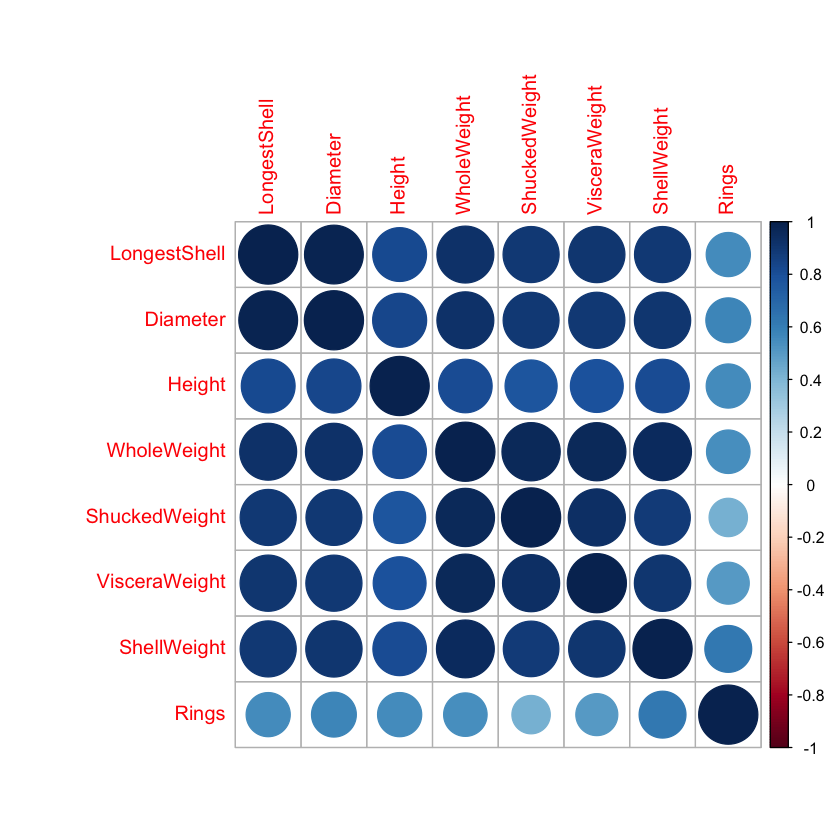# Abalone Prediction in R¶

In :
# Abalone

# Background
# Dataset description: http://archive.ics.uci.edu/ml/datasets/Abalone

# 0. Load Libraries
library(AppliedPredictiveModeling)
library(caret)
library(doMC)
library(corrplot)
registerDoMC(cores=4)

# 1. Load Dataset
data(abalone)
dataset <- abalone

# 2. Summarize Dataset
dim(dataset)
# list types for each attribute
sapply(dataset, class)
# split input and output
x <- dataset[,1:8]
y <- dataset[,9]
# summarize attribute distributions
summary(dataset)
# summarize correlations between input variables
cor(x[,2:8])

# 3. Visualize Dataset
# a) Univariate
# boxplots for each attribute
par(mfrow=c(3,3))
for(i in 2:9) {
boxplot(dataset[,i], main=names(dataset)[i])
}
# histograms each attribute
par(mfrow=c(3,3))
for(i in 2:9) {
hist(dataset[,i], main=names(dataset)[i])
}
# density plot for each attribute
par(mfrow=c(3,3))
for(i in 2:9) {
plot(density(dataset[,i]), main=names(dataset)[i])
}

par(mfrow=c(1,1))
# b) Multivariate
# scatterplot matrix
pairs(dataset)

# correlation plot
correlations <- cor(dataset[,2:9])
corrplot(correlations, method="circle")

# 4. Feature Selection
# a) remove redundant
# b) remove highly correlated

# 5. Data Transforms

# 6. Evaluate Algorithms
control <- trainControl(method="repeatedcv", number=10, repeats=3)
metric <- "RMSE"
seed <- 6

# a) linear algorithms
# GLM
set.seed(seed)
fit.glm <- train(Rings~., data=dataset, method="glm", metric=metric, trControl=control)
print(fit.glm)

# lm
set.seed(seed)
fit.lm <- train(Rings~., data=dataset, method="lm", metric=metric, trControl=control)
print(fit.lm)

# b) nonlinear algorithms
# SVM
set.seed(seed)
fit.svm <- train(Rings~., data=dataset, method="svmRadial", metric=metric, trControl=control)
print(fit.svm)

# CART
set.seed(seed)
fit.cart <- train(Rings~., data=dataset, method="rpart", metric=metric, trControl=control)
print(fit.cart)

# kNN
set.seed(seed)
fit.knn <- train(Rings~., data=dataset, method="knn", metric=metric, trControl=control)
print(fit.knn)

# c) advanced algorithms
# Bagging
set.seed(seed)
fit.bagging <- train(Rings~., data=dataset, method="treebag", metric=metric)
print(fit.bagging)

set.seed(seed)
fit.boosting <- train(Rings~., data=dataset, method="gbm", metric=metric, trControl=control)
print(fit.boosting)

1. 4177
2. 9
Type
'factor'
LongestShell
'numeric'
Diameter
'numeric'
Height
'numeric'
WholeWeight
'numeric'
ShuckedWeight
'numeric'
VisceraWeight
'numeric'
ShellWeight
'numeric'
Rings
'integer'
 Type      LongestShell      Diameter          Height        WholeWeight
F:1307   Min.   :0.075   Min.   :0.0550   Min.   :0.0000   Min.   :0.0020
I:1342   1st Qu.:0.450   1st Qu.:0.3500   1st Qu.:0.1150   1st Qu.:0.4415
M:1528   Median :0.545   Median :0.4250   Median :0.1400   Median :0.7995
Mean   :0.524   Mean   :0.4079   Mean   :0.1395   Mean   :0.8287
3rd Qu.:0.615   3rd Qu.:0.4800   3rd Qu.:0.1650   3rd Qu.:1.1530
Max.   :0.815   Max.   :0.6500   Max.   :1.1300   Max.   :2.8255
ShuckedWeight    VisceraWeight     ShellWeight         Rings
Min.   :0.0010   Min.   :0.0005   Min.   :0.0015   Min.   : 1.000
1st Qu.:0.1860   1st Qu.:0.0935   1st Qu.:0.1300   1st Qu.: 8.000
Median :0.3360   Median :0.1710   Median :0.2340   Median : 9.000
Mean   :0.3594   Mean   :0.1806   Mean   :0.2388   Mean   : 9.934
3rd Qu.:0.5020   3rd Qu.:0.2530   3rd Qu.:0.3290   3rd Qu.:11.000
Max.   :1.4880   Max.   :0.7600   Max.   :1.0050   Max.   :29.000  
LongestShellDiameterHeightWholeWeightShuckedWeightVisceraWeightShellWeight
LongestShell1.00000000.98681160.82755360.92526120.89791370.90301770.8977056
Diameter0.98681161.00000000.83368370.92545210.89316250.89972440.9053298
Height0.82755360.83368371.00000000.81922080.77497230.79831930.8173380
WholeWeight0.92526120.92545210.81922081.00000000.96940550.96637510.9553554
ShuckedWeight0.89791370.89316250.77497230.96940551.00000000.93196130.8826171
VisceraWeight0.90301770.89972440.79831930.96637510.93196131.00000000.9076563
ShellWeight0.89770560.90532980.81733800.95535540.88261710.90765631.0000000Generalized Linear Model

4177 samples
8 predictor

No pre-processing
Resampling: Cross-Validated (10 fold, repeated 3 times)
Summary of sample sizes: 3759, 3759, 3759, 3759, 3760, 3760, ...
Resampling results:

RMSE      Rsquared  MAE
2.213138  0.530665  1.585958

Linear Regression

4177 samples
8 predictor

No pre-processing
Resampling: Cross-Validated (10 fold, repeated 3 times)
Summary of sample sizes: 3759, 3759, 3759, 3759, 3760, 3760, ...
Resampling results:

RMSE      Rsquared  MAE
2.213138  0.530665  1.585958

Tuning parameter 'intercept' was held constant at a value of TRUE
Support Vector Machines with Radial Basis Function Kernel

4177 samples
8 predictor

No pre-processing
Resampling: Cross-Validated (10 fold, repeated 3 times)
Summary of sample sizes: 3759, 3759, 3759, 3759, 3760, 3760, ...
Resampling results across tuning parameters:

C     RMSE      Rsquared   MAE
0.25  2.181121  0.5634193  1.482162
0.50  2.153219  0.5696081  1.470037
1.00  2.142313  0.5711541  1.466745

Tuning parameter 'sigma' was held constant at a value of 0.259324
RMSE was used to select the optimal model using the smallest value.
The final values used for the model were sigma = 0.259324 and C = 1.

Warning message in nominalTrainWorkflow(x = x, y = y, wts = weights, info = trainInfo, :
“There were missing values in resampled performance measures.”
CART

4177 samples
8 predictor

No pre-processing
Resampling: Cross-Validated (10 fold, repeated 3 times)
Summary of sample sizes: 3759, 3759, 3759, 3759, 3760, 3760, ...
Resampling results across tuning parameters:

cp          RMSE      Rsquared   MAE
0.03890424  2.626421  0.3370765  1.949066
0.05432313  2.701929  0.2974648  2.004954
0.28217437  2.996754  0.2543435  2.205786

RMSE was used to select the optimal model using the smallest value.
The final value used for the model was cp = 0.03890424.
k-Nearest Neighbors

4177 samples
8 predictor

No pre-processing
Resampling: Cross-Validated (10 fold, repeated 3 times)
Summary of sample sizes: 3759, 3759, 3759, 3759, 3760, 3760, ...
Resampling results across tuning parameters:

k  RMSE      Rsquared   MAE
5  2.239805  0.5211464  1.575896
7  2.197834  0.5379101  1.534888
9  2.188602  0.5420856  1.526652

RMSE was used to select the optimal model using the smallest value.
The final value used for the model was k = 9.
Bagged CART

4177 samples
8 predictor

No pre-processing
Resampling: Bootstrapped (25 reps)
Summary of sample sizes: 4177, 4177, 4177, 4177, 4177, 4177, ...
Resampling results:

RMSE      Rsquared   MAE
2.272856  0.4995728  1.622254

Iter   TrainDeviance   ValidDeviance   StepSize   Improve
1        9.6453             nan     0.1000    0.7458
2        9.0169             nan     0.1000    0.5774
3        8.5173             nan     0.1000    0.5141
4        8.1018             nan     0.1000    0.4200
5        7.7435             nan     0.1000    0.3702
6        7.4350             nan     0.1000    0.3005
7        7.1538             nan     0.1000    0.2685
8        6.9395             nan     0.1000    0.2059
9        6.7364             nan     0.1000    0.2027
10        6.5644             nan     0.1000    0.1652
20        5.5219             nan     0.1000    0.0408
40        4.8348             nan     0.1000    0.0059
60        4.5171             nan     0.1000    0.0099
80        4.3371             nan     0.1000    0.0032
100        4.2196             nan     0.1000   -0.0021
120        4.1315             nan     0.1000   -0.0031
140        4.0683             nan     0.1000    0.0002
150        4.0236             nan     0.1000   -0.0020

4177 samples
8 predictor

No pre-processing
Resampling: Cross-Validated (10 fold, repeated 3 times)
Summary of sample sizes: 3759, 3759, 3759, 3759, 3760, 3760, ...
Resampling results across tuning parameters:

interaction.depth  n.trees  RMSE      Rsquared   MAE
1                   50      2.438257  0.4390650  1.760440
1                  100      2.342626  0.4810819  1.686715
1                  150      2.288431  0.5026718  1.646601
2                   50      2.280865  0.5085633  1.636033
2                  100      2.186541  0.5425262  1.552927
2                  150      2.166855  0.5492125  1.533952
3                   50      2.218790  0.5317232  1.578110
3                  100      2.166761  0.5493806  1.531263
3                  150      2.156756  0.5534389  1.521503

Tuning parameter 'shrinkage' was held constant at a value of 0.1

Tuning parameter 'n.minobsinnode' was held constant at a value of 10
RMSE was used to select the optimal model using the smallest value.
The final values used for the model were n.trees = 150, interaction.depth =
3, shrinkage = 0.1 and n.minobsinnode = 10.In [ ]: